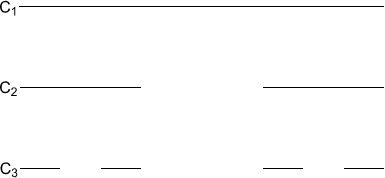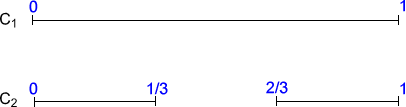#### You may also like### Tweedle Dum and Tweedle Dee

Two brothers were left some money, amounting to an exact number of pounds, to divide between them. DEE undertook the division. "But your heap is larger than mine!" cried DUM...### Sum Equals Product

The sum of the numbers 4 and 1 [1/3] is the same as the product of 4 and 1 [1/3]; that is to say 4 + 1 [1/3] = 4 ï¿½ 1 [1/3]. What other numbers have the sum equal to the product and can this be so for any whole numbers?### Special Sums and Products

Find some examples of pairs of numbers such that their sum is a factor of their product. eg. 4 + 12 = 16 and 4 × 12 = 48 and 16 is a factor of 48.

# The Cantor Set

##### Age 11 to 14Challenge Level

Take a line segment of length 1. We'll call it $C_1$.

Now remove the middle third. Call what's left $C_2$.

Now remove the middle third of each line segment in $C_2$. Call what's left $C_3$.

We can keep doing this, at each stage removing the middle third of each of the line segments in $C_n$ to form $C_{n+1}$.Draw pictures of $C_4$ and $C_5$.

If we suppose that the end points of $C_1$ are 0 and 1, then we can mark on the end points of the line segments for the later $C_n$ too. For example, $C_2$ has end points $0$, $\frac{1}{3}$, $\frac{2}{3}$ and $1$ as shown below.Draw $C_3$ and label the end points, and label the end points on your pictures of $C_4$ and $C_5$.

We can keep removing middle thirds infinitely many times. The set of points left having done it infinitely many times is called the Cantor set.

Which of the following points are in the Cantor set?

$\frac{1}{3}$, $\frac{4}{9}$, $\frac{3}{81}$, $\frac{4}{81}$.

Explain how you decided which belong and which don't.